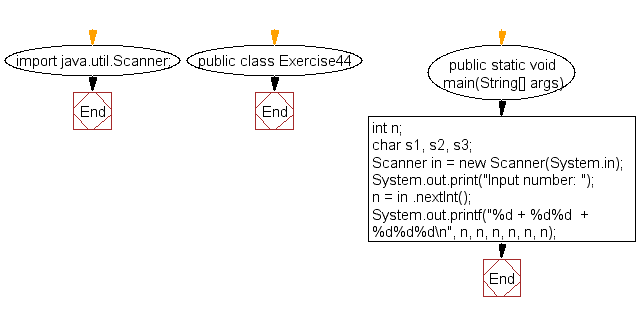﻿ Java: Computes the value of n + nn + nnn# Java Exercises: Accepts an integer (n) and computes the value of n+nn+nnn

## Java Basic: Exercise-44 with Solution

Write a Java program that accepts an integer (n) and computes the value of n+nn+nnn.

Sample Solution:

Java Code:

``````import java.util.Scanner;
public class Exercise44 {
public static void main(String[] args) {

int n;
char s1, s2, s3;
Scanner in = new Scanner(System.in);
System.out.print("Input number: ");
n = in .nextInt();
System.out.printf("%d + %d%d  + %d%d%d\n", n, n, n, n, n, n);
}
}
```
```

Sample Output:

```Input number: 5
5 + 55  + 555
```

Flowchart:Java Code Editor:

What is the difficulty level of this exercise?

Test your Programming skills with w3resource's quiz.

﻿

## Java: Tips of the Day

getEnumMap

Converts to enum to Map where key is the name and value is Enum itself.

```public static <E extends Enum<E>> Map<String, E> getEnumMap(final Class<E> enumClass) {
return Arrays.stream(enumClass.getEnumConstants())
.collect(Collectors.toMap(Enum::name, Function.identity()));
}
```

Ref: https://bit.ly/3xXcFZt